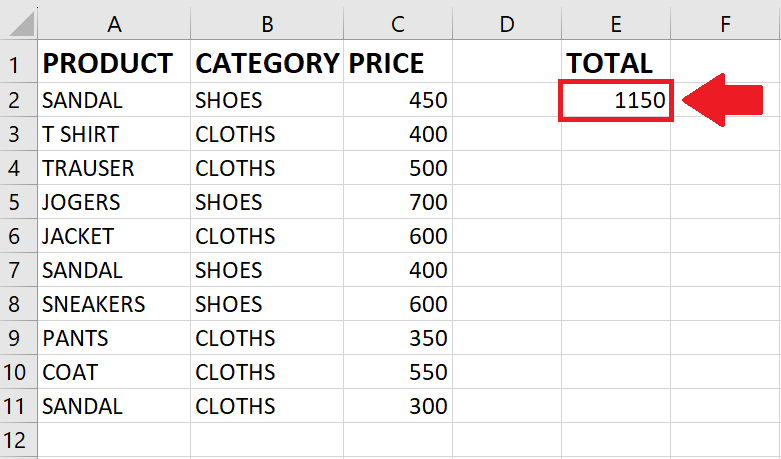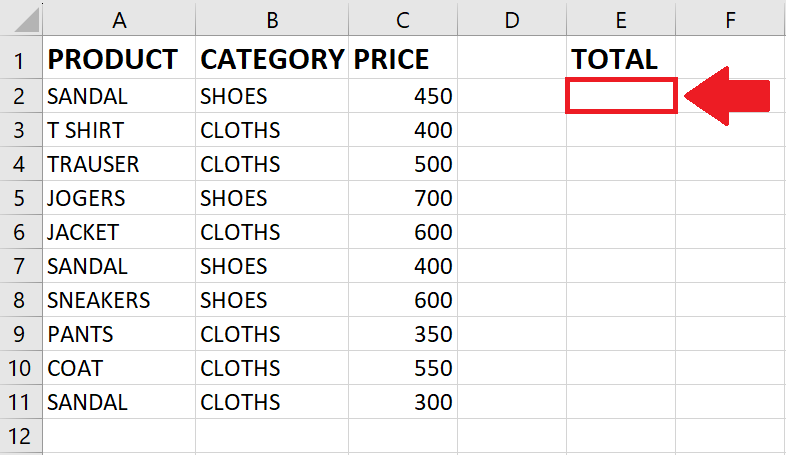# How to use sumifs not equal in Excel

In this tutorial, we will learn how to use the SUMIFS function with not equal conditions. We have a dataset containing a list of products and their prices, categorized into clothes and shoes. Our goal is to calculate the total price specifically for sandals using the SUMIFS function.

The SUMIFS function is a powerful tool in Excel used for summing values that meet multiple criteria. It allows you to specify conditions or criteria for different ranges and then calculates the sum of values that satisfy all the specified conditions. The general syntax of the SUMIFS function is as follows:

=SUMIFS(sum_range, criteria_range1, criteria1, [criteria_range2, criteria2], …)

sum_range refers to the range of cells that contain the values you want to sum.

criteria_range1 is the range of cells to which you want to apply the first criterion to.

criteria1 is the condition or value that you want to use as the first criterion.

[criteria_range2, criteria2] (optional) represents additional ranges and criteria that you can include for more specific conditions.In Excel, the SUMIFS function is used to calculate the sum of values in a range that meets multiple criteria. It allows you to specify multiple conditions and sum the values that satisfy all those conditions. When you use the SUMIFS function with the “not equal to” criteria, you can sum the values that do not meet a specific condition. In other words, it allows you to exclude certain values from the sum based on a particular criterion.

### Step 1 – Select the Cell– Click on the cell where you want to show the result

### Step 2 – Use the SUMIF function– After selecting the cell, click on the SUMIF function
=SUMIFS(

### Step 3 – Type the Arguments of the function– After using the function, type the Arguments as follows

=SUMIFS(C2:C11,B2:B11,”<>CLOTHES”,A2:A11,”SANDAL”)

– After typing the arguments, type the closing bracket
– This formula sums the values in the range C2:C11 (prices) where the corresponding cells in B2:B11 (categories) are not equal to “CLOTHES” and the corresponding cells in A2:A11 (products) are equal to “SANDAL”.

### Step 4 – Press the Enter key– After typing the arguments, press the Enter key to get the required result